﻿ 基于局部均值分解和相关向量机的变形预测文章快速检索 高级检索
 大地测量与地球动力学2018, Vol. 38Issue (11): 1128-1132  DOI: 10.14075/j.jgg.2018.11.006### 引用本文LUO Yiyong, XU Zhikuan, ZHANG Liting, et al. Deformation Prediction Based on Local Mean Decomposition and Relevance Vector Machine[J]. Journal of Geodesy and Geodynamics, 2018, 38(11): 1128-1132.### Foundation support

National Natural Science Foundation of China, No.41861058;Key Laboratory for Digital Land and Resources of Jiangxi Province, East China University of Technology, No. DLLJ201612; Science and Technology Project of the Education Department of Jiangxi Province, No. GJJ150592.

### About the first author

LUO Yiyong, PhD candidate, associate professor, majors in deformation data processing method, E-mail:luoyiyong@whu.edu.cn.

### 文章历史

1. 东华理工大学测绘工程学院，南昌市广兰大道418号，330013;
2. 武汉大学测绘学院，武汉市珞喻路129号，430079;
3. 东华理工大学江西省数字国土重点实验室，南昌市广兰大道418号，330013

1 LMD和RVM算法的改进 1.1 LMD算法的改进 1.1.1 LMD基本原理

 $\left\{ \begin{array}{l} {u_1}\left( t \right) = x\left( t \right) - {\rm{P}}{{\rm{F}}_1}\left( t \right)\\ {u_2}\left( t \right) = {u_1}\left( t \right) - {\rm{P}}{{\rm{F}}_2}\left( t \right)\\ \;\;\;\;\;\;\;\;\;\;\;\;\; \vdots \\ {u_k}\left( t \right) = {u_{k - 1}}\left( t \right) - {\rm{P}}{{\rm{F}}_q}\left( t \right) \end{array} \right.$ (1)

 $x\left( t \right) = \mathop \sum \limits_{q = 1}^k {\rm{P}}{{\rm{F}}_q}\left( t \right) + {u_k}\left( t \right)$ (2)
1.1.2 LMD算法的改进

1.2 RVM算法的改进 1.2.1 RVM基本原理

 $\alpha _i^{{\rm{opt}}} = \frac{{1 - {\alpha _i}{S_{i, i}}}}{{F_i^2}}$ (3)
 ${({\sigma ^2})^{{\rm{opt}}}} = \frac{{{{\left| {\left| {t - \mathit{\Phi }F} \right|} \right|}^2}}}{{N - \mathop \sum \limits_{i = 0}^N (1 - {\alpha _i}{S_{i, i}})}}$ (4)

1.2.2 RVM算法的改进

 $\begin{array}{l} K = \mu \exp \left( { - \frac{{||{x_i} - {x_j}|{|^2}}}{{{\psi ^2}}}} \right) + \\ \;\;\;\;\;\left( {1 - \mu } \right){\left[ {{x_i}{{\left( {\frac{{{x_j}}}{{{\chi ^2}}}} \right)}^{\rm{T}}} + 1} \right]^2} \end{array}$ (5)

1.3 模型建立结构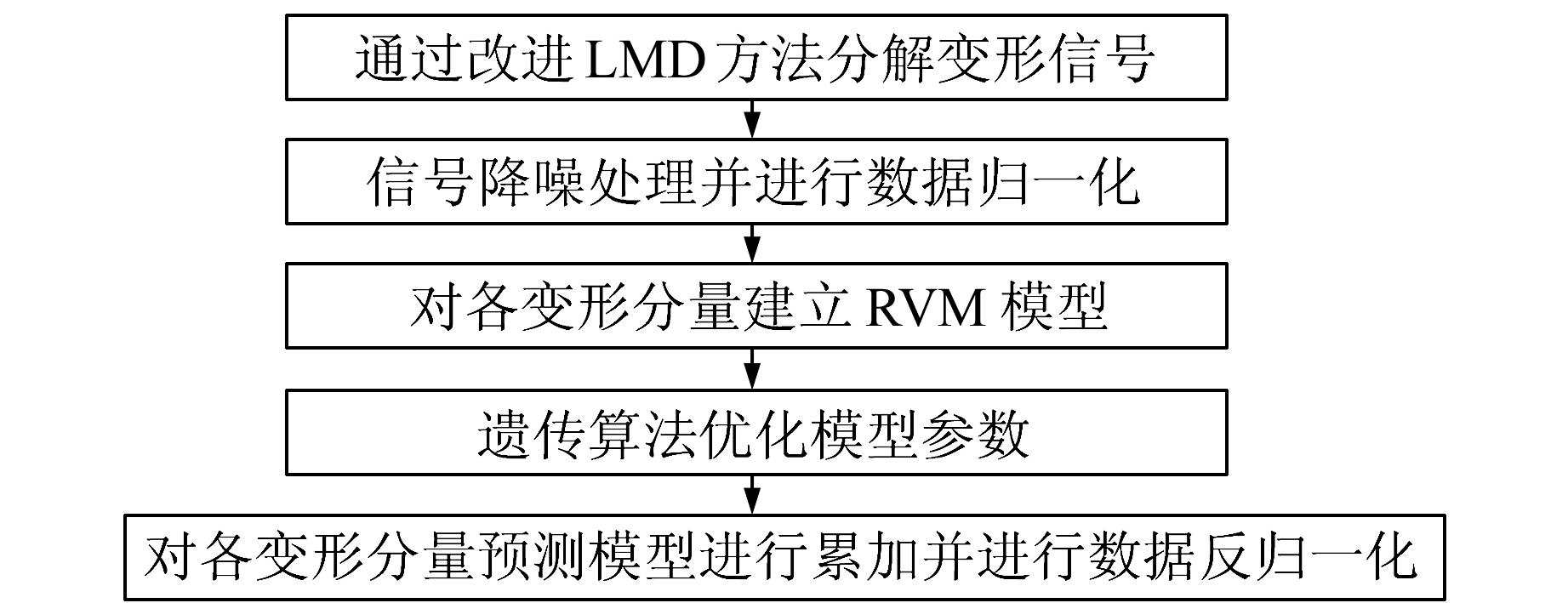图 1 改进LMD-RVM预测模型结构图 Fig. 1 Sketch of improved LMD-RVM's prediction model

2 基于改进LMD-RVM模型的大坝变形预测

2.1 LMD处理大坝监测数据及小波阈值去噪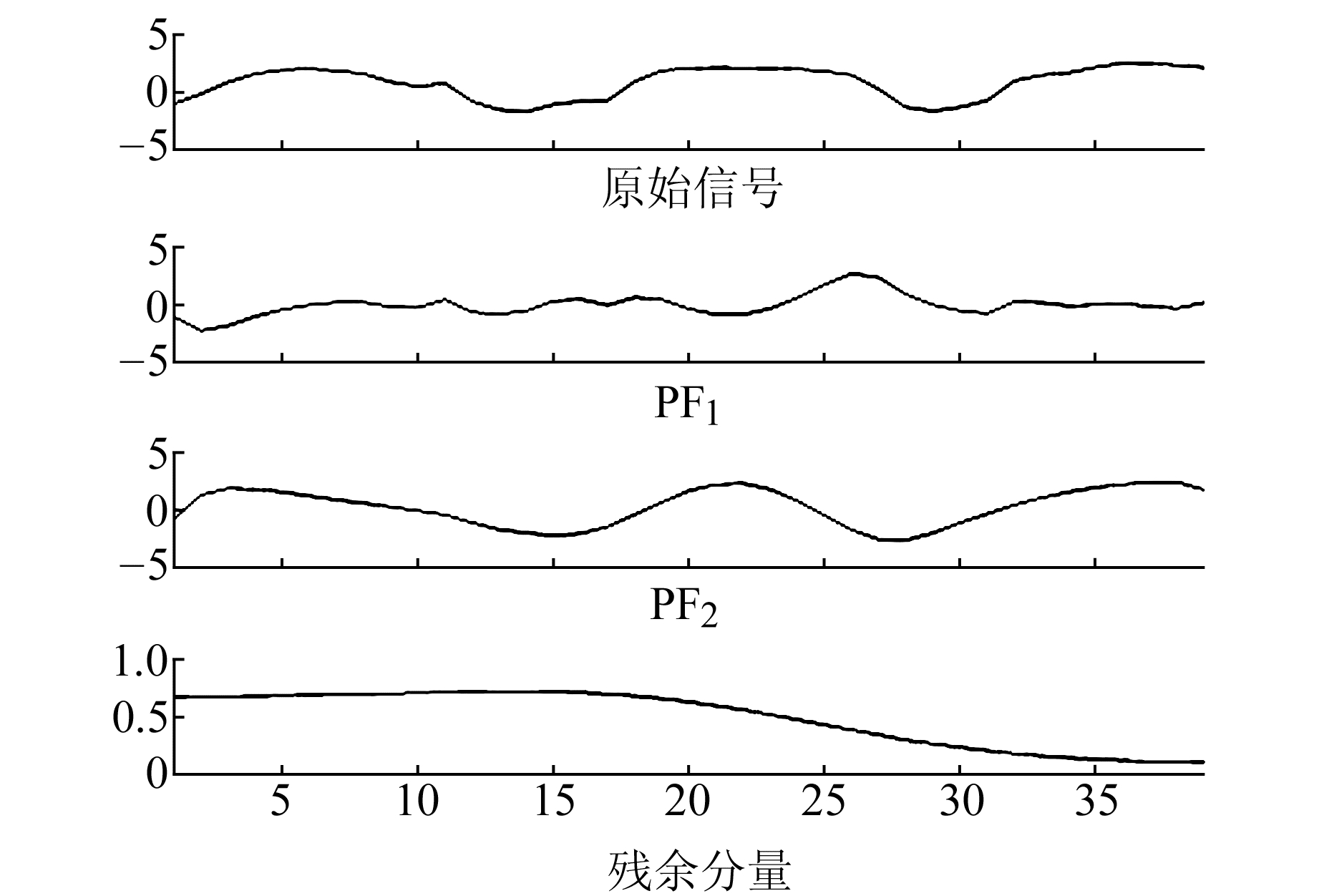图 2 改进LMD分解后变形分量 Fig. 2 Deformation components of improved LMD

2.2 RVM模型预测结果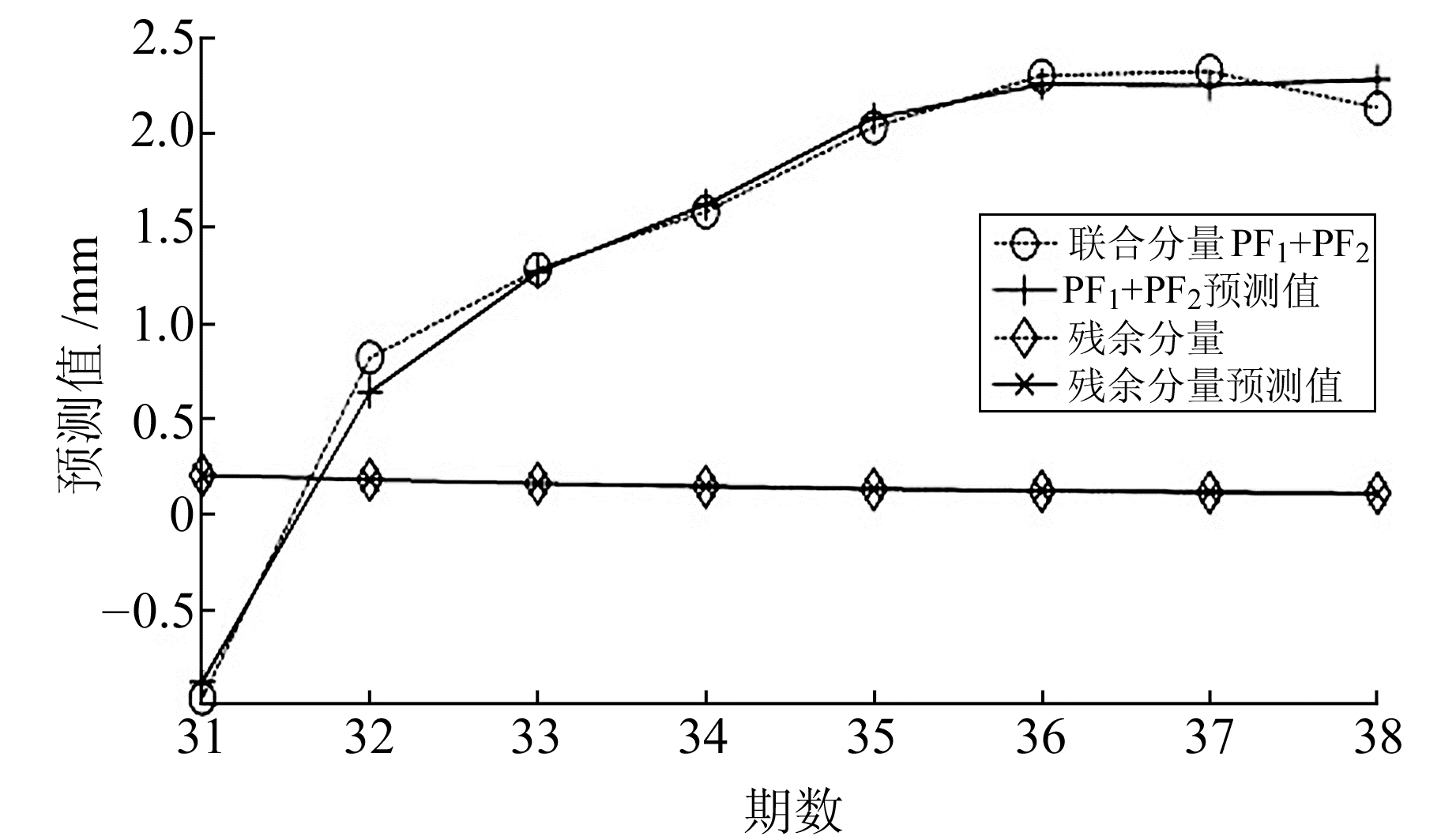图 3 各变形分量预测结果 Fig. 3 The prediction results of each deformation component

3 精度分析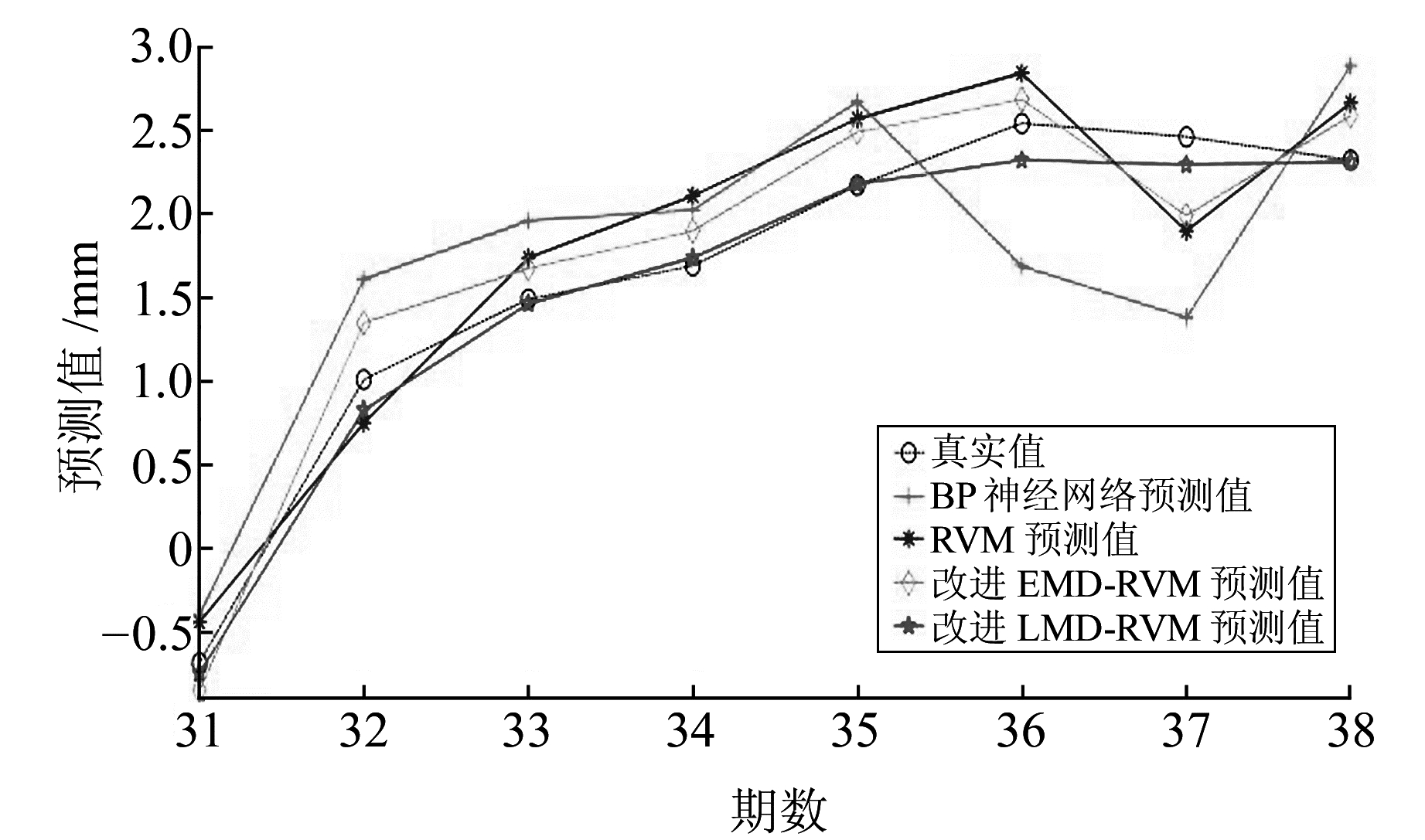图 4 各方法预测曲线图和实际变形量 Fig. 4 The curves of each method predict and actual deformation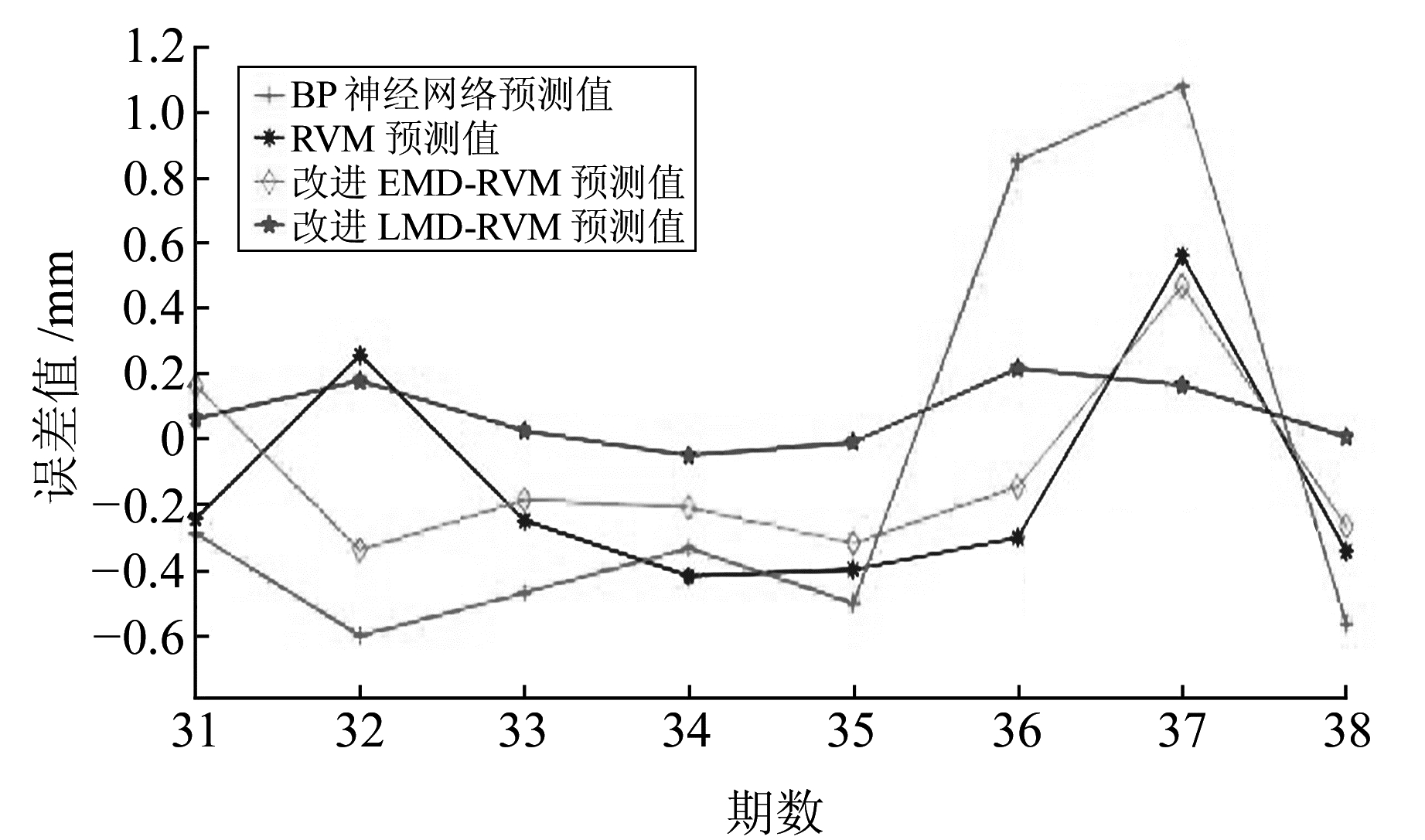图 5 各方法预测值绝对误差 Fig. 5 The absolute error of each method predict value表 1 测试数据预测结果及精度评价指标 Tab. 1 Predictive results and accuracy evalution index of test data

4 结语

1) 通过改进LMD自适应分解非平稳信号获得多个具有一定物理特征的变形分量，能有效处理干扰因素对数据的影响，能适当减少模型结构的复杂程度且对挖掘数据内部规律有一定提升。

2) 建立RVM预测模型在处理小样本、贫信息及复杂非线性问题等方面具有很大优势，同时该方法求得的多项精度指标值均优于BP神经网络方法和RVM方法的预测结果，证实该方法具有较好的鲁棒性和较强的泛化能力，是一种预测精度较高的方法。

  刘娜, 栾元重, 黄晓阳, 等. 基于时间序列分析的桥梁变形监测预报研究[J]. 测绘科学, 2011, 36(6): 46-48 (Liu Na, Luan Yuanzhong, Huang Xiaoyang, et al. Bridge Deformation Monitoring and Forecasting Based on Time Series Analysis[J]. Science of Surveying and Mapping, 2011, 36(6): 46-48) (0)  靖洪文, 吴俊浩, 马波, 等. 基于模糊灰色系统的深部巷道围岩变形预测模型及应用[J]. 煤炭学报, 2012(7): 1099-1104 (Jing Hongwen, Wu Junhao, Ma Bo, et al. Prediction Model and Its Application of Deep Mine Tunnel Surrounding Rock Deformation Based on Fuzzy-Grary System[J]. Journal of China Coal Society, 2012(7): 1099-1104) (0)  刘贺, 张弘强, 刘斌. 基于粒子群优化神经网络算法的深基坑变形预测方法[J]. 吉林大学学报:地球科学版, 2014(5): 1609-1614 (Liu He, Zhang Hongqiang, Liu Bin. A Prediction Method for the Deformation of Deep Foundation Pit Based on the Particle Swarm Optimization Neural Network[J]. Journal of Jilin University:Earth Science Edition, 2014(5): 1609-1614) (0)  谭衢霖, 魏健, 胡吉平. 基于小波神经网络的建筑工程沉降变形预测[J]. 应用基础与工程科学学报, 2015(3): 629-636 (Tan Qulin, Wei Jian, Hu Jiping. Building Deformation Prediction Based on Wavelet Analysis and BP Neural Network[J]. Journal of Basic Science and Engineering, 2015(3): 629-636) (0)  赵艳南, 牛瑞卿, 彭令, 等. 基于粗糙集和粒子群优化支持向量机的滑坡变形预测[J]. 中南大学学报:自然科学版, 2015(6): 2324-2332 (Zhao Yannan, Niu Ruiqing, Peng Ling, et al. Prediction of Landslide Deformation Based on Rough Sets and Particle Swarm Optimization-Support Vector Machine[J]. Journal of Central South University:Science and Technology, 2015(6): 2324-2332) (0)  许同乐, 王营博, 郑店坤, 等. 基于LMD-ICA降噪的滚动轴承故障特征提取方法研究[J]. 北京邮电大学学报, 2017(1): 111-116 (Xu Tongle, Wang Yingbo, Zheng Diankun, et al. Research of the Rolling Bearing Fault Signal Feature Extraction Method Based on the LMD-ICA Noise Reduction[J]. Journal of Beijing University of Posts and Telecommunications, 2017(1): 111-116) (0)  王建国, 陈帅, 张超. 噪声参数最优ELMD与LS-SVM在轴承故障诊断中的应用与研究[J]. 振动与冲击, 2017(5): 72-78 (Wang Jianguo, Chen Shuai, Zhang Chao. Application of Noise Parametric Optimization with ELMD and LS-SVM in Bearing Fault Diagnosis[J]. Journal of Vibration and Shock, 2017(5): 72-78) (0)  马春辉, 杨杰. 基于KPCA-RVM的土石坝沉降预测模型研究[J]. 西北农林科技大学学报:自然科学版, 2017(1): 211-217 (Ma Chunhui, Yang Jie. KPCA-RVM Based Prediction Model for Settlement of Earth-Rockfill Dam[J]. Journal of Northwest A & F University:Nat Sci Ed, 2017(1): 211-217) (0)  Zhao W G, Gao Y F, Li C L. RVM Based on PSO for Groundwater Level Forecasting[J]. Journal of Computers, 2012, 7(5): 1073-1079 (0)  郭航忠, 丛培江. Hibert-Huang变换在大坝监测资料分析中的应用[J]. 武汉大学学报:信息科学版, 2007, 32(9): 774-777 (Guo Hangzhong, Cong Peijiang. Application of Hibert-Huang Transform in Analysis on Monitoring Data of Dam[J]. Geomatics and Information Science of Wuhan University, 2007, 32(9): 774-777) (0)  王婷. EMD算法研究及其在信号去噪中的应用[D].哈尔滨: 哈尔滨工程大学, 2010 (Wang Ting. Research on EMD Algorithm and Its Application in Signal Denoising[D]. Harbin: Harbin Engineering University, 2010) http://cdmd.cnki.com.cn/Article/CDMD-10217-1011020271.htm (0)  秦国华, 何志芬, 王华敏, 等. 基于动力学与遗传算法的工件位置偏离预测与控制方法[J]. 机械工程学报, 2017(1): 110-120 (Qin Guohua, He Zhifen, Wang Huamin, et al. Prediction and Control of Workpiece Position Deviation Based on Dynamics and Genetic Algorithm[J]. Journal of Mechanical Engineering, 2017(1): 110-120) (0)
Deformation Prediction Based on Local Mean Decomposition and Relevance Vector Machine
LUO Yiyong1,2,3XU Zhikuan1ZHANG Liting1     HUANG Xiaolang1     MIAO Yuzhou1
1. Faculty of Geomatics, East China University of Technology, 418 Guanglan Road, Nanchang 330013, China;
2. School of Geodesy and Geomatics, Wuhan University, 129 Luoyu Road, Wuhan 430079, China;
3. Key Laboratory for Digital Land and Resources of Jiangxi Province, East China University of Technology, 418 Guanglan Road, Nanchang 330013, China
Abstract: A new multi-scale deformation prediction method is proposed based on improved local mean decomposition (LMD) and weighted kernel function relevance vector machine algorithm (RVM). The deformation data is decomposed into several physical deformation components by LMD, and each deformation component is predicted by RVM optimized by genetic algorithm. Finally, a multi-scale deformation prediction method is established and applied to dam deformation prediction by adding the prediction results of each deformation component. The experimental results show that the improved LMD-RVM method is superior to the BP neural network method, RVM method and the improved EMD-RVM method in many precision indexes, which proves the effectiveness and reliability of the new method.
Key words: local mean decomposition; relevance vector machine; deformation prediction